# Embedded System to Detect Wildfire

Creating a system in order to detect wildfire using deep learning programmation on a Raspberry Pi 4 and a Wisol module by Sigfox.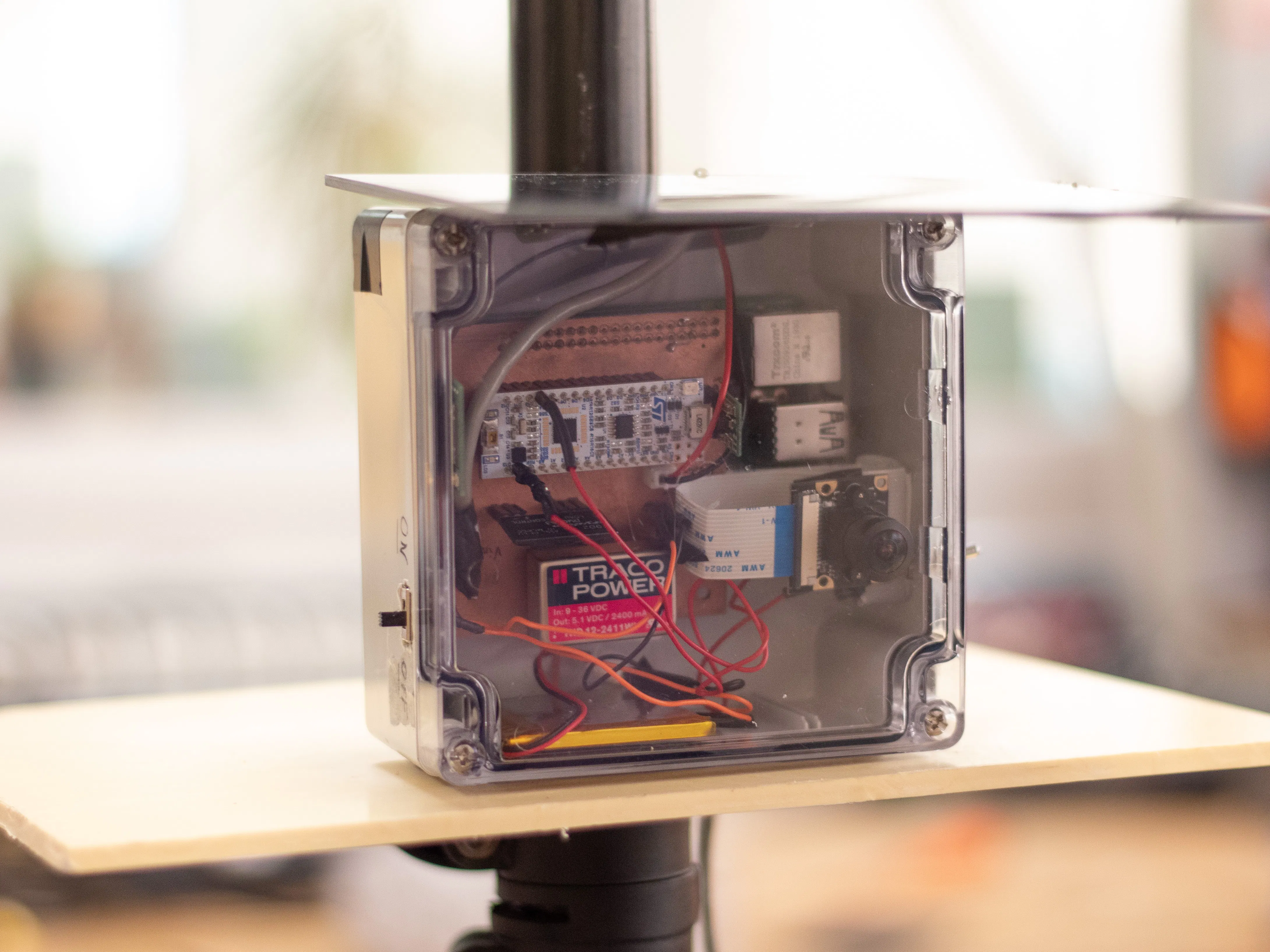## Things used in this project

### Hardware components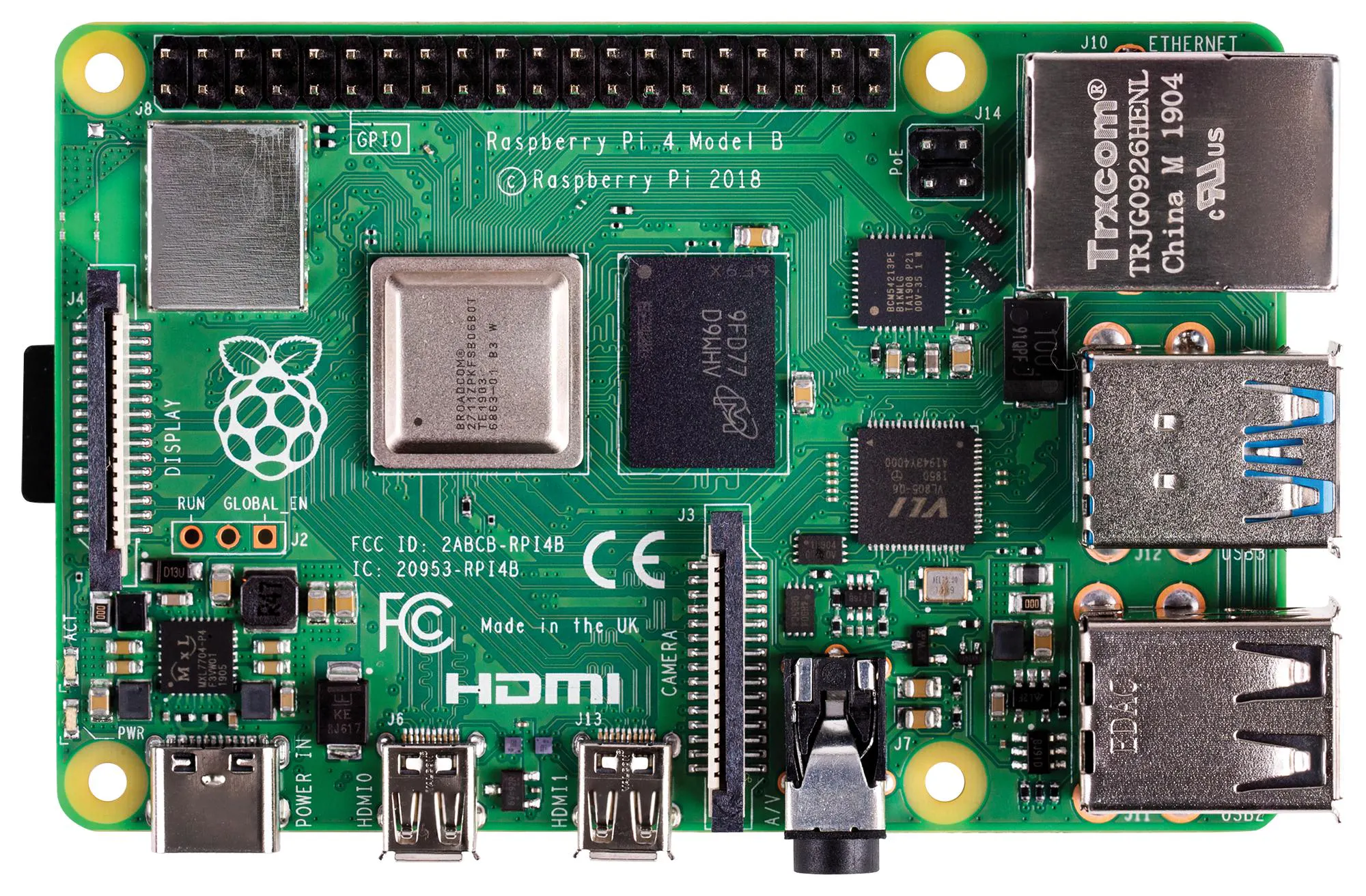Raspberry Pi 4 Model B
×1
 module camera 5Mpx for Raspberry Pi You can find it also here : https://www.generationrobots.com/fr/403500-module-caméra-grand-angle-pour-raspberry-pi-et-nvidia-jetson-nano.html?search_query=Module+Camera&results=60
×1
 ST STM32L4, STM32F7 Microcontroller Nucleo STM32L432KC You can find it also here : https://www.mouser.fr/ProductDetail/STMicroelectronics/NUCLEO-L432KC?qs=qzCNEk%252BRr%252BajjPvxjwWK5g==&vip=1&gclid=CjwKCAiA6bvwBRBbEiwAUER6JdeExAEDAjNMAm7sxNf_B5uRvyP7MMzrZfKAjjIoJdSaM38VsGYKpBoCQfIQAvD_BwE
×1
 Sigfox Wisol Module
×1
 Molex Patch Antenna Flexible
×1
 MikroElektronika Solar Cell You can also find it here : https://fr.rs-online.com/web/p/panneaux-solaires/8829073
×1
×1
 DC-DC Converter Step-Down (Out 5,1V)
×1
 DC-DC Converter Step-Down / Step-Up Pololu (Out : 3.3V 1A)
×1
 Static Relay (Out : 3A)
×1
 SparkFun LiPo Rider Board
×1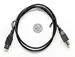USB-A to B Cable
×1
 Micro USB-B Converter
×1×1Resistor 1k ohm
×3
 Resistor 2k ohm
×1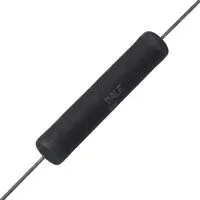Through Hole Resistor, 4.02 kohm
×1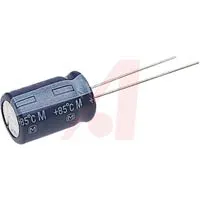Capacitor 4.7 µF
×2
 Microchip Charger LiPo Battery (CMS)
×1
 Rohm Resistor 1k ohm (CMS)
×3
 Rohm Resistor 2k ohms (CMS)
×1
 Resistor 4.02k ohms (CMS)
×1
 Capacitor 4.7 uF
×1
×1

## Code

### RaspfireDetection

C/C++
```#include "mbed.h"

Timer t;
Timer t2;
Timer t3;
InterruptIn raspi(D3);
DigitalOut interrupteur(D13);
Serial sig(D5,D4);
Serial pc(SERIAL_TX, SERIAL_RX);
DigitalIn MODE(D9);
float tps = 0;

void start_Timer(){
t.start();
}

void stop_Timer(){
t.stop();
t.reset();
}

void nivBat(){
float VMaxBattRPI = 13.5;
float VMinBattRPI = 11;
float VMaxLipo = 4;
float VMinLipo = 3;
int rasp;
int bat;
float VLipoPercent = ((VLipoReal - VMinLipo)*100)/(VMaxLipo - VMinLipo);
float VbattRPIPercent = ((VbattRPIReal - VMinBattRPI)*100)/(VMaxBattRPI - VMinBatt            RPI);

if(VbattRPIPercent < 0.0){
rasp = 0;
}
if(VLipoPercent < 0.0){
bat = 0;
}
if(VbattRPIPercent > 100.0){
rasp = 100;
}
if(VLipoPercent > 100.0){
bat = 100;
}
if(VLipoPercent >= 0.0 && VLipoPercent <= 100.0){
bat = (int)(VLipoPercent);
}
if(VbattRPIPercent >= 0.0 && VbattRPIPercent <= 100.0){
rasp = (int)(VbattRPIPercent);
}
else{
bat = 222;
rasp = 222;
}
sig.printf("AT\$SF=%02x%02x%02x\r\n",0, bat, rasp);
pc.printf("AT\$SF=%02x%02x%02x\r\n",0, bat, rasp);
}

int main() {
if(MODE){ //Mode Demo
raspi.rise(&start_Timer);
raspi.fall(&stop_Timer);
int a = 1;
int b = 0;
int c = 2;
int d = 3;
int level = 0;
pc.printf("mode DEMO");
nivBat();
while (true) {
interrupteur = 1;
t2.start();
if(tps <= 0.30  && tps >= 0.10 && level == 0){
t2.reset();
sig.printf("AT\$SF=%02x%02x%02x\r\n", a, 222, 222);
pc.printf("AT\$SF=%02x%02x%02x\r\n", a, 222, 222);
sig.printf("AT\$SF=%02x%02x%02x\r\n", b, 222, 222);
pc.printf("AT\$SF=%02x%02x%02x\r\n", b, 222, 222);
t.reset();
tps = 0;
wait(5);
++level;
}
else if(tps <= 0.30  && tps >= 0.10 && level == 1){
sig.printf("AT\$SF=%02x%02x%02x\r\n", c, 222, 222);
pc.printf("AT\$SF=%02x%02x%02x\r\n", c, 222, 222);
sig.printf("AT\$SF=%02x%02x%02x\r\n", b, 222, 222);
pc.printf("AT\$SF=%02x%02x%02x\r\n", b, 222, 222);
t.reset();
tps = 0;
wait(5);
++level;
}
else if(tps <= 0.30  && tps >= 0.10 && level == 2){
sig.printf("AT\$SF=%02x%02x%02x\r\n", d, 222, 222);
pc.printf("AT\$SF=%02x%02x%02x\r\n", d, 222, 222);
sig.printf("AT\$SF=%02x%02x%02x\r\n", b, 222, 222);
pc.printf("AT\$SF=%02x%02x%02x\r\n", b, 222, 222);
t.reset();
++level;
tps = 0;
t.reset();
wait(1);
interrupteur = 0;
wait(5);
interrupteur = 1;
}
level = 0;
t2.reset();
nivBat();
}
}
}

else{ //Mode normal
WakeUp::calibrate();
raspi.rise(&start_Timer);
raspi.fall(&stop_Timer);
int a = 1;
int b = 0;
int c = 2;
int d = 3;
int level = 0;
pc.printf("mode Normal");
nivBat();
while (true) {
interrupteur = 1;
t2.start();
if(tps <= 0.30  && tps >= 0.10 && level == 0){
t2.reset();
sig.printf("AT\$SF=%02x%02x%02x\r\n", a, 222, 222);
pc.printf("AT\$SF=%02x%02x%02x\r\n", a, 222, 222);
sig.printf("AT\$SF=%02x%02x%02x\r\n", b, 222, 222);
pc.printf("AT\$SF=%02x%02x%02x\r\n", b, 222, 222);
t.reset();
tps = 0;
wait(10);
++level;
}
else if(tps <= 0.30  && tps >= 0.10 && level == 1){
sig.printf("AT\$SF=%02x%02x%02x\r\n", c, 222, 222);
pc.printf("AT\$SF=%02x%02x%02x\r\n", c, 222, 222);
sig.printf("AT\$SF=%02x%02x%02x\r\n", b, 222, 222);
pc.printf("AT\$SF=%02x%02x%02x\r\n", b, 222, 222);
t.reset();
tps = 0;
wait(10);
++level;
}
else if(tps <= 0.30  && tps >= 0.10 && level == 2){
sig.printf("AT\$SF=%02x%02x%02x\r\n", d, 222, 222);
pc.printf("AT\$SF=%02x%02x%02x\r\n", d, 222, 222);
sig.printf("AT\$SF=%02x%02x%02x\r\n", b, 222, 222);
pc.printf("AT\$SF=%02x%02x%02x\r\n", b, 222, 222);
t.reset();
tps = 0;
t.reset();
wait(1);
interrupteur = 0;
wait(10);
interrupteur = 1;
}
level = 0;
interrupteur = 0;
wait(36000);
interrupteur = 1;
float VMaxBattRPI = 13.5;
float VMinBattRPI = 11;
float VMaxLipo = 4;
float VMinLipo = 3;
float VLipoPercent = ((VLipoReal - VMinLipo)*100)/(VMaxLipo - VMinLipo);
float VbattRPIPercent = ((VbattRPIReal - VMinBattRPI)*100)/(VMaxBattRPI - VMinBattRPI);
int rasp;
int bat;
if(VbattRPIPercent < 0.0){
rasp = 0;
}
if(VLipoPercent < 0.0){
bat = 0;
}
if(VbattRPIPercent > 100.0){
rasp = 100;
}
if(VLipoPercent > 100.0){
bat = 100;
}
if(VLipoPercent >= 0.0 && VLipoPercent <= 100.0){
bat = (int)(VLipoPercent);
}
if(VbattRPIPercent >= 0.0 && VbattRPIPercent <= 100.0){
rasp = (int)(VbattRPIPercent);
}
else{
bat = 222;
rasp = 222;
}
sig.printf("AT\$SF=%02x%02x%02x\r\n",0, bat, rasp);
t2.reset();
}
}
}
}
```

## Credits

### enzo calvino

1 project • 2 followers

### Audrey Delorme

1 project • 2 followers
Élève ingénieure en Electronique, Informatique et Systemes embarqués - BAC+4

### minngie bartnik

1 project • 2 followers

### Mickael Ramilison

1 project • 2 followers
Thanks to Mr Yann Douze and Mr Sylvain Viateur.